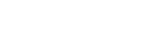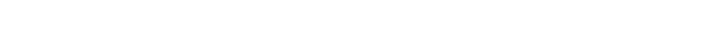# Potential Transformer and its Application Explained

## What is a Potential Transformer

Potential Transformer is an instrument transformer that is used for the voltage transformation from a greater value to the lesser. Therefore, it is a step-down transformer which lowers the voltage to a safer limit. This lower voltage is measured easily by any low voltage instrument like wattmeter, voltmeter, etc,.

A potential transformer is also called a voltage transformer. These are designed for measuring high voltage connected to the transmission and distribution systems. The voltage transformer steps down the voltage level and makes it easier to calculate the voltage value with a simple low voltage instrument.

In other words, the measuring instruments are at low voltage so cannot be connected directly to the transmission and distribution lines. Thus, are transformed into lower values and measured. Also, these transformers work as an isolator for the circuit. It protects the measuring circuit from the mainline (circuit) which works at a higher level of voltage.

## Potential Transformer Construction

The voltage transformer is a step-down transformer. Therefore, the number of primary turns (winding) is but has more turns with a lesser number of turns at secondary. At the input of the transformer, high voltage AC is given. After transformation, the lower voltage is obtained at the output of the potential transformer. This output voltage is measured with the help of a voltmeter. Also, the two primary and secondary windings are electrically isolated and mechanically coupled.

Note: An AC voltage is converted to DC by a half-wave rectifier and/or a full-wave bridge rectifier.Potential Transformer

Voltage transformers are constructed in such a way that it can operate at lower magnetic current, flux density, and at minimized load. The conductors used here are large and the core is made from iron. Mostly the core type potential transformer is used, though both shell type and core type can be designed easily.

Since the primary voltage is quite high, therefore, it is divided into smaller sections (turns/ratio). This reduces the cost of insulation and the risk of damaging.  One more important factor while construction of the potential transformer is the phase shift between the primary and the secondary winding. It is carefully observed to have a lower voltage by varying the load.

### Connections in PT

The potential transformer always connects in parallel with the mains (transmission and distribution circuits). The primary of the voltage transformer directly connects to the mainline. The voltage of the mains is then calculated. Measuring instruments like a voltmeter or wattmeter connected to the secondary output of the transformer. Therefore, the high line voltage is measured with a lot of ease with such low voltages measuring devices.

Since the transformer connection is mechanically coupled and electrically isolated, therefore, the circuit is completely safe and also the measuring is done properly. Also, the primary of the voltage transformer is rated for 400 volts to thousands of volts. The secondary is rated for max 400 volts.

## Potential Transformer Working Principle

The principle of the potential transformer is based on mutual induction. The connection of the power circuit to the voltage transformer is between phase and ground. The primary and secondary winding of the transformer is magnetically coupled through the minimum reluctance path of the core (but electrically isolated).

Now, the input of the transformer is given with the high voltage which produces a flux. This flux traverse through the core and induces the voltage at the secondary winding of the transformer. Therefore, through the principle of electromagnetic induction, the high voltage is converted to a lower voltage. This happens without any physical connection between the two.

Also, as the potential transformer has a larger value of impedance, therefore, the smaller value of current flows through the secondary winding. The other function of this transformer is similar to that of the ordinary transformer.

### Equivalent Circuit of the Potential Transformer

The equivalent circuit of the voltage transformer is the same as the ordinary one.Equivalent circuit of Potential Transformer

Where,

Vp = source voltage/primary voltage

N1 = primary turns

N2 = secondary turns

Vs = secondary voltage

I1 = primary current

I2 = secondary current

R1 = leakage resistance of the primary

X1 = leakage reactance of the primary

Xm = magnetic reactance

R2 = leakage resistance of the primary

X2 = leakage reactance of the primary

The rest of the calculations is the same as for the ordinary transformer.

## Types of Potential Transformer

These transformers are categorized based on their function as well as on their construction.

Depending on the construction the transformer can be classified as the

• Metering voltage transformers
• Protection voltage transformers

Both of these are available in both single-phase and three-phase and have good accuracy. The use of such transformers is to control and operate measuring devices like relays, etc,.

Based on construction, potential transformers can be classified as “Electromagnetic type” and “Capacitive type”. Let us discuss these in detail.

### Electromagnetic Type Potential Transformer

This type of potential transformer is similar to the wire wound oil-filled conventional transformers. The tap tank is connected to the line terminal. To fill oil, a plug is there at the tank. It is minted on the insulator support.

In this type of PT, the primary winding connects between the two phases. Or between a phase and the ground. Therefore, the one end of the primary is connected to the mains (of the measuring power circuit). And the other to the ground (common ground terminal).

The secondary of the transformer is given to the load, i.e., to the multimeter or the voltmeter to observe the voltage value. Once the value is calculated at the secondary then with the use of turns ratio, we can calculate the voltage of the higher primary side. The following formula is useful for the calculation:With the knowledge of all the parameters, we calculate the value of V2.

The secondary of this transformer is also useful in the protection of the circuit in case of faults. Therefore, relays can serve the purpose.

### Capacitive Potential Transformer

A voltage transformer is a capacitive voltage divider. It is connected between the phase of the mains and the ground. It can either be a coupling type or a bushing type. The main difference between the two is in the way in which capacitance is formed. This, therefore, helps in determining the rating of the burden.Capacitor Potential Transformer

This type of PT has a series of capacitors connected. All these coupling capacitors are made of either aluminum foil or oil-impregnated paper. The connection of primary and secondary across these capacitors give the desirable voltage values on both sides.

When compared with the electromagnetic type, this capacitive voltage transformer is more economical.

## Errors in Potential or Voltage Transformer

The error means the difference observed in the true and the measured value. In an ideal transformer, the voltage produced in the secondary is in exact proportion to that of the primary. Ideally, this voltage ratio is equal to the ratio of the number of wounds (turns) on the primary to that on the secondary winding.

But practically, in potential transformers, these are not achievable. This is because of the primary and secondary voltage drops (due to resistances and reactances) and also due to the power factor of the load at secondary. This creates errors in the system. Two types of errors can be there, the ratio and the phase angle error. Let us discuss both the errors in detail.

### Phase Angle Error

Ideally, the phase angle difference should be zero between the primary and the secondary voltages. But practically, it does not happen, and we have a phase difference. This difference in phase is the phase angle error.

To reduce the resistance and the reactance of the transformer should be optimum. Also, the no-load current plays a role in making or breaking the phase angle error.

### Ratio Error

The ratio error is the difference in the measured value from the actual one (ratio). Therefore, if it occurs in the transformer, then the voltage at the secondary deviates from the true value. Following is the formula for the ratio error calculation:### Reducing Errors in Voltage Transformer

The following points help reduce the error in the potential transformer:

• By reducing the distance between the primary and the secondary. It reduces the leakage reactance and hence the error reduces.
• Providing high flux densities (in the core) and reducing the resistance of the winding. To achieve this we reduce the length of the wound winding over the core.
• By reducing the length of the mean turn of the windings and the use of thick conductors.
• Reduce the primary no-load current of the transformer.
• Reduce the length of the magnetic path (for flux) in the core.

## Applications of Potential Transformer

We have immense applications of potential transformers. Following are the few applications:

• In Electrical protection
• In metering circuits and relay
• Used in protecting feeders
• For synchronizing generators with grid
• In impedance protection of generators
• Used in protection voltage transformers
• As carrier communication circuitsVoltage Transformer

The voltage transformer used for measurement is called a measuring potential transformer. While transformers useful for protection of power circuits are known as the voltage protection transformers. Similarly, there are cases when we want both measurement and protection. Therefore, at secondary one connects for protection, and the other connects to the metering for measurement.

## Characteristics of Potential Transformer

Let us now see what effect comes when we do slight changes in parameters at the secondary of the potential transformers.

### Effect of Power Factor Change at Secondary Side

When the power factor of the load at the secondary of the transformer reduces then the phase angle increases. Therefore, the primary current goes equal to the no-load current and the primary and the secondary voltages are in phase with induced voltage in both primary and secondary.

Thus, the voltage at the primary remains the same. But the transformation ratio increases. This is because of the decrease in power factor. So note the following points as follows:

• Ratio error decreases with a decrease in the power factor at the secondary side.
• Phase angle error increases with the decreasing power factor.

### Effect of Voltage Change at Secondary Side

As the voltage at the load side increases, consequently the secondary current increases. This increase in the secondary current increases the primary current. Hence, the voltage drops due to resistance and reactance increases.

These voltage drops in both primary and secondary decreases the voltage at the load terminal. Therefore, this increases the transformation ratio as the denominator decreases. Please note the following points when secondary voltage changes :

• With an increase in the voltage at the secondary of the potential transformer, the ratio error also increases.
• If we draw a graph between the phase angle error and the secondary voltage, then we get a linear curve. Therefore, the increase or decrease of the voltage will increase or decrease the phase angle error respectively.

So we can say that the load voltage is directly proportional to both the errors, ratio, and phase angle error.